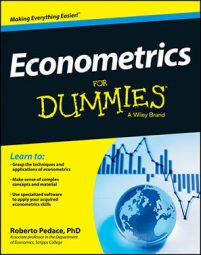##### Econometrics For DummiesMany economic phenomena are dichotomous in nature; in other words, the outcome either occurs or does not occur. Dichotomous outcomes are the most common type of discrete or qualitative dependent variables analyzed in economics. For example, a student who applies to graduate school will be admitted or not. If you're interested in determining which factors contribute to graduate school admission, then your outcome or dependent variable is dichotomous.

As with outcomes that are quantitative, regression models can be used to explain the occurrence of these qualitative events.,They usually require special treatment, however, because they're likely to create some problems for traditional regression analysis. Econometricians have developed techniques to address those issues. The techniques can be quantitatively burdensome and practically impossible without a computer, but understanding the structure of the models is essential if you want to accurately interpret the computer output.

Like qualitative variables, when quantitative variables with restricted values are used as dependent variables, they also generate unique circumstances for regression analysis. The problem, as is the case with qualitative variables, is that the limited (censored or truncated) values cause the distributional assumptions of the classical linear regression model to fail. Suppose you're interested in estimating the effect of price changes on the demand for U2 concert tickets. One problem is that the measurement of this demand is limited by the capacity of the arenas booked for their tour. After a concert sells out, you may not be able to observe by how much the demand exceeded the arena limit. You may only observe limited values for many quantitative variables (for example, various types of percentages, SAT scores, grade point averages, and so on).

Fortunately, econometricians have developed techniques to handle restricted/limited dependent variables that are similar to those used for qualitative dependent variables.

The following list contains special dependent variable situations and the names of the techniques econometricians have developed to handle them:

• Dichotomous or binary response dependent variable: A discrete variable with two outcomes, usually 0 or 1. Handled with Probit/Logit models.

• Censored dependent variable: A continuous variable where some of the actual values have been limited to some predetermined minimum or maximum value. Handled with the Tobit (censored normal) model.

• Truncated dependent variable: A continuous variable where some of the actual values aren't observed if they are less than some predetermined minimum value or more than some predetermined maximum value. Handled with the truncated normal model.

• Self-selected sample: Missing values for the dependent variable due to nonrandom participation decisions from population of interest. Handled with the Heckman selection model.

• Polychotomous or a multiple response dependent variable: A discrete variable with more than two outcomes. Handled with a multinomial Probit/Logit model or ordered Probit/Logit model (covered in more advanced econometrics courses).

• Discrete dependent variable: A nonnegative, discrete count variable that assumes integer values (0, 1, 2,…). Handled with a Poisson model or negative binomial model (covered in more advanced econometrics courses).